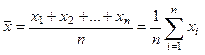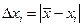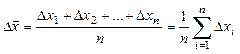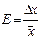# Principal concepts of the theory of errors

We can't define the true values of a physical quantity. We can define only the interval (xmin, xmax) of the investigated quantity with some probability a. For example: we can affirm, that students' height may be defined between 1.5 m and 2.0 m with probability of 0.9. Then we can prove, that students' height may be defined between 1.6 m and 1.8 m with smaller probability of 0.6 and so on. Value of this interval is called the entrusting interval. On fig.2.1 interval of quantity being investigated x is represented.Figure 2.1

Where x is the most probable value of quantity being measured; Dx is the half width of the entrusting interval of the measured quantity with probability of a.

Therefore we can estimate, that true value of the measured quantity may be defined as x = xD x, with probability a,

or.

If a quantity x has been measured n times and x1 , x2 ,..., xn are the results of the individual measurements then the most probable measured value or the arithmetic mean is:(2.1)

The deviationis called the accidental error (deviation) of a single measurement.(2.2)

is called the mean accidental deviation of the measurements.

Mean root square is defined as(2.3)

where t – Student’s constant for definite a and n. The ratio of(2.4)

is called the relative error of measurement and is usually expressed in percents:. (2.5)

Поможем в написании учебной работы
Поможем с курсовой, контрольной, дипломной, рефератом, отчетом по практике, научно-исследовательской и любой другой работой

 Дата добавления: 2015-08-12; просмотров: 240. Нарушение авторских прав; Мы поможем в написании вашей работы!
Studopedia.info - Студопедия - 2014-2022 год . (0.02 сек.) русская версия | украинская версия
Поможем в написании
> Курсовые, контрольные, дипломные и другие работы со скидкой до 25%
3 569 лучших специалисов, готовы оказать помощь 24/7